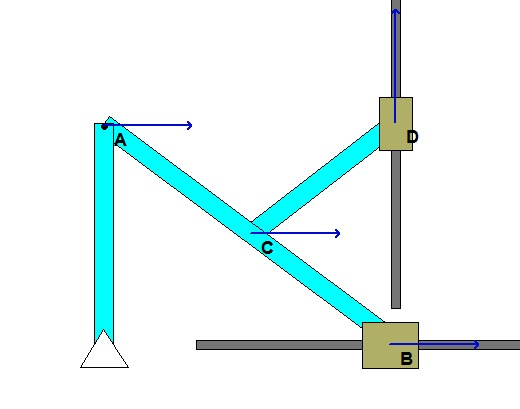# Homework H.3.E# Homework H.3.F# Homework H.3.CDiscussionShown above is the motion of the mechanism as seen by a fixed observer.For the same motion, the above animation shows the view of an observer that is attached to the slotted link.

It is suggested that you employ the view of the observer on the slotted arm in your analysis.

# Homework H.3.D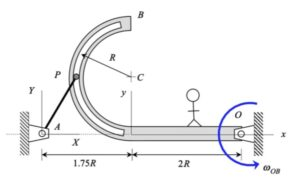Discussion• Shown above left is the motion of the mechanism as seen by a fixed observer. Note that the stationary observer sees P moving on a circular path centered at point A.
• And, shown above right is the motion of P as seen by an observer attached to the slotted arm. Note that the moving observer sees P moving on a circular path centered at point C.

It is suggested that you employ the view of the observer on the slotted arm in your analysis.

# Homework H.3.ADiscussionAn animation from the simulation of the motion of the robotic arm system is shown above for a fixed observer. The motion of end-effector B is quite complicated, as can be seen. However, if we attach an observer to section AD of the end link of the arm, the observed motion of B is greatly simplified. In fact, the observer simply sees a back-and-forth motion of B in the x-direction, as shown in the animation below.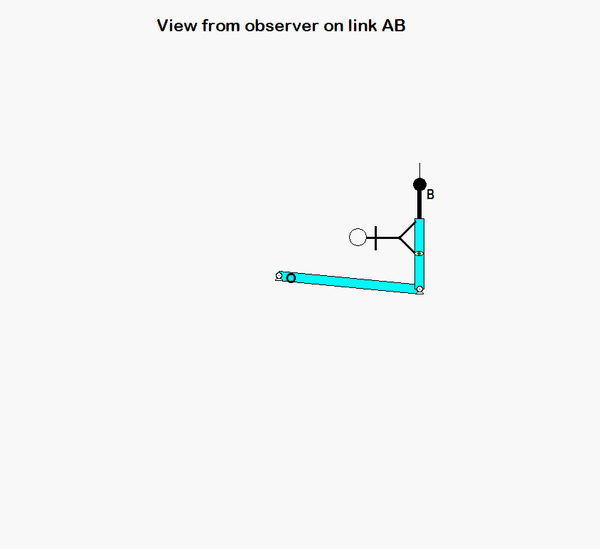HINTS: The velocity of point B can be found from the following moving reference frame velocity equation:

vB = vA + (vB/A)rel + ω × rB/A

vA = (L θ_dot) i
ω
= φ_dot k
(vB/A)rel = b_dot i

Use similar logic for writing down the acceleration of B. Be careful in writing down the expression for aA ; in particular, a0. (A is traveling on a circular path with a constant speed.)

# Homework H.3.B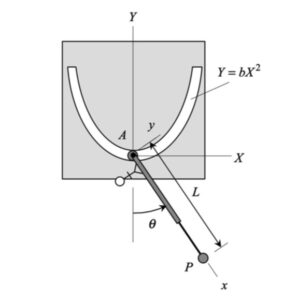# Homework H.2.I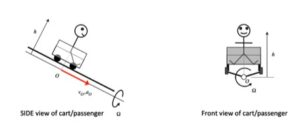# Homework H.2.JDiscussionThe motion of this four-bar mechanism is quite complicated. However, we can make some sense out of the velocities of points B and D by using instant centers (ICs). For a given position of the mechanism, can you visualize the location of the IC for link BD? And from this location, do the directions for the velocities for points B and D make sense?

HINTS:
You are asked to perform the complete kinematical analysis for the mechanism for the position shown below.

Velocity
For the velocity analysis, you are encouraged to use the IC center approach. Where is the IC for link BD located? What does the location of this IC say about the angular velocity of link BD? Use that result to determine the angular velocity of link DE.

Acceleration
This is a standard kinematics of a mechanism problem. For this, consider the following steps:

1. Write down the acceleration of point B using link AB.
2. Write down the acceleration expression for D using link DE.
3. Write down the acceleration expression for B using link DB and your result for the acceleration of D from Step 2.
4. Equate the expressions for the acceleration of B in Steps 1 and 3 above. From this, solve for the angular accelerations of links BD and DE.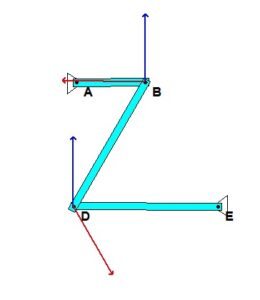# Homework H.2.GDiscussion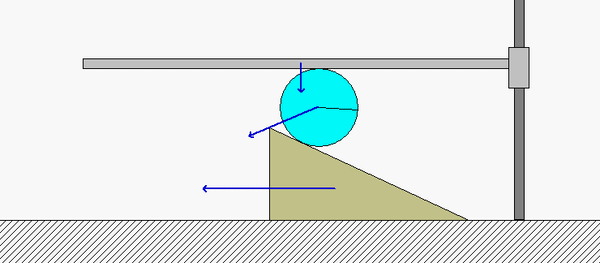Points B and D on the disk roll without slipping on the horizontal guide A and the wedge E, respectively. Because of this:

• B has a velocity vB which points straight down, with the same speed vA as the horizontal guide A.
• D has a velocity vD which points directly to the left with the same speed as the wedge E.

The instant center (IC) of the disk (call it point C) therefore exists at the intersection of the perpendiculars to vand vD. Note that point B is much closer to the IC than point D. What does this say about the relative sizes of vD and vB? Is this consistent with the speeds of D and B shown in the above animation?

HINTS:

• Use the above discussion points to locate the IC for the disk.
• Determine the angular speed of the disk based on the speed of B and the distance from B to C.
• The speed of the wedge E is found by using the angular speed from above and the distance from C to D.

# Homework H.2.HDiscussion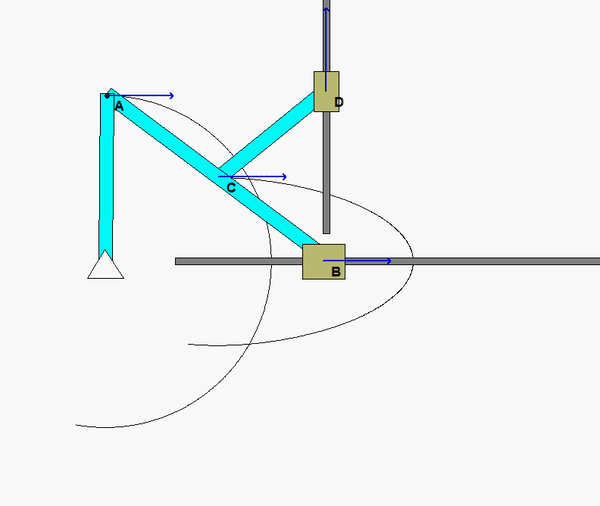The animation above shows the motion of the mechanism over a range of input angles of link OA. For a given position, envision the location of the instant centers (ICs) for links AB and CD. Do the directions and magnitudes for the velocities of points B, C and D agree with the location of these ICs?

Shown below if a freeze-frame of the mechanism motion at the position for which you are asked to do analysis. From this figure, where are the two ICs for links CD and BC? In particular, how does the position of the IC for AB relate to the relative sizes of the speeds of points A, C and B? What is the angular velocity of link AB at this position?